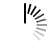# C# Linq FirstorDefault Method

Use the FirstorDefault() method to return the first element of a sequence or a default value if element isn’t there.

The following is our empty list −

```List<double> val = new List<double> { };
```

Now, we cannot display the first element, since it is an empty collection. For that, use the FirstorDefault() method to display the default value.

```val.AsQueryable().FirstOrDefault();
```

The following is the complete example.

## Example

Live Demo

```using System;
using System.Collections.Generic;
using System.Linq;
class Demo {
static void Main() {
List<double> val = new List<double> { };
double d = val.AsQueryable().FirstOrDefault();
Console.WriteLine("Default Value = "+d);
if (d == 0.0D) {
d = 0.1D;
}
Console.WriteLine("Default Value changed = "+d);
}
}
```

## Output

```Default Value = 0
Default Value changed = 0.1
```